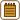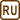## Magnetic Force between Two Wires Carrying Current

### Task number: 2112

The distance between two parallel wires carrying currents of 10 A and 20 A is 10 cm. Determine the magnitude and direction of the magnetic force acting on the length of 1 m of wires, if the currents are carried a) in the same direction, b) in the opposite direction.

• #### Hint

Draw the magnetic field which the wire with current creates around itself.

Since one of the wires is located in the magnetic field of the other one, it will be influenced by the magnetic force whose magnitude is proportional to the magnitude of the magnetic field caused by the second wire, to the magnitude of the current in the first wire, and to the length of the wire. The direction of this force can be determined by the Fleming's left-hand rule.

• #### Analysis

Each wire carrying electric current creates a magnetic field around itself. We can draw this field after determining the direction of the magnetic field lines using the Ampère's right-hand grip rule.

Each wire also lies in the magnetic field of the other wire. Since the wires carry electric current and are located in a magnetic field, they are under the influence of a magnetic force. Magnitude of the magnetic force depends on the magnitude of the magnetic field, current carried by the wire, length of the wire and on the wire's position in relation to the vector of the magnetic field. Since the wires are parallel to each other, the direction of the magnetic field is going to be perpendicular to the direction of current in the wire (see more detailed explanation in the Hint section).

Magnetic field in which one of the wires is located is created by the other wire. We substitute for the magnitude of the field of a long straight wire (in this case, of the second wire) into the relation for the magnitude of the magnetic field. The distance at which we want to determine the magnitude of the magnetic field is equal to the distance between the wires.

We can apply a similar logic to the other wire, which is located in the magnetic field of the first one. It is also influenced by the magnetic force of the first wire.

Directions of the forces with which the wires are acting on each other can be determined by the Fleming's left-hand rule.

• #### Solution of a): Congruent direction of the current

Each wire carrying electric current creates a magnetic field around itself. We can determine the direction of the magnetic field by the Ampère's right-hand grip rule.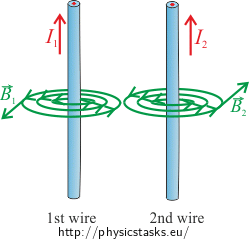One wire lies in the magnetic field of the other wire, thus there is a magnetic force acting on the first wire, for which the following holds true:

$F_2=B_2I_1l\tag{1}$

where B2 is the magnitude of the magnetic field created by the second wire in the place in which the first wire is located, I1 is the magnitude of the current carried by the first wire.

For the magnitude of the magnetic field B2 we have

$B_2= \frac{\mu_o}{2\pi}\,\frac{I_2}{R}$

where R is the distance from the place in which we are determining the magnitude of the magnetic field. In our case, it is the place where the second wire is. Thus, R is the distance between the wires.

We substitute the magnitude of the magnetic field B2 in the formula for the magnitude of the magnetic force F2 and adjust the formula:

$F_2=\frac{\mu_o}{2\pi}\,\frac{I_1I_2}{R}l.$

We have obtained the relation for the magnitude of the magnetic force F2 by which the second wire affects the first one.

Direction of the magnetic force can be determined by the Fleming’s left-hand rule. In our case, magnetic force $$\vec{F}_2$$ is directed to the right.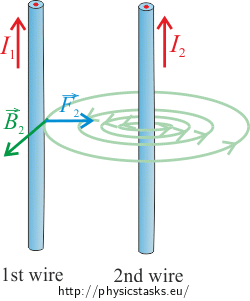Note: For the sake of clarity, the picture shows only the magnetic field created by the second wire, as the magnetic force of the second wire $$\vec{F}_2$$ influences the first wire exactly because of it.

Likewise, the second wire is located in the magnetic field of the first wire, therefore, it is also influenced by a magnetic force. For the magnitude of this force it holds true:

$F_1=B_1I_2l,$

where B1 is the magnitude of the magnetic field of the first wire and I2 is the magnitude of the current carried by the second wire.

For the magnitude of the magnetic field B1 we have:

$B_1= \frac{\mu_o}{2\pi}\,\frac{I_1}{R}.$

Then we substitute for this expression into the formula for the magnitude of the magnetic force F1

$F_1=\frac{\mu_o}{2\pi}\,\frac{I_1I_2}{R}l.$

Direction of the magnetic force $$\vec{F}_1$$ can also be determined by the Fleming's left-hand rule. In our case, the force with which the first wire influences the second one is directed to the left.Magnitudes of the forces $$\vec{F}_1$$ and $$\vec{F}_2$$ with which the wires are acting on each other are equal. Let's denote this common value as F.

$F_1=F_2=F=\frac{\mu_o}{2\pi}\,\frac{I_1I_2}{R}l.$

So, wires carrying current are acting on each other by the forces of the same magnitude, but the opposite direction. If we remember the formulation of the Newton's third law (the action-reaction law), then we could come to this conclusion directly from the third law without determining the direction and magnitude of the second force.

If the direction of the current carried by the wires is congruent, then the wires are attracted to each other.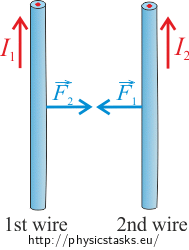• #### Solution of b): Opposite direction of the current

Around each wire carrying electric current, a magnetic field will arise, and the direction of this field can be determined by the Ampère's right-hand grip rule.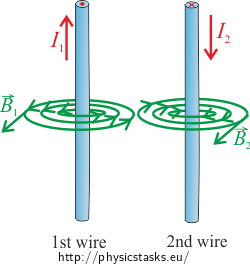If we change the direction of the current in the wire, only the direction of the magnetic field and the direction of the force will change. Yet the magnitude of the magnetic force acting on the wires will be the same as in case of the congruent direction of the current. For the magnitude of the magnetic force acting on each wire the relation derived in Solution of a) can be applied:

$F=\frac{\mu_o}{2\pi}\,\frac{I_1I_2}{R}l.$

Direction of the magnetic force acting on the first wire can be determined by the Fleming's left-hand rule. In our case, the magnetic force with which the second wire influences the first one is directed to the left.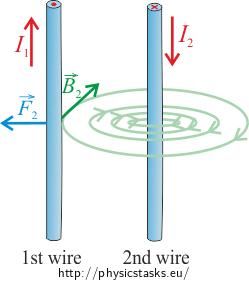Direction of the magnetic force acting on the second wire can also be determined by the Fleming's left-hand rule. In our case, the magnetic force with which the first wire influences the second one is directed to the right.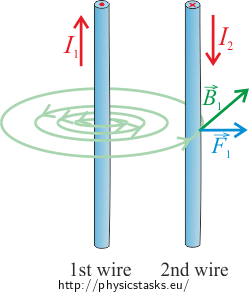So, wires carrying current are acting on each other by the forces of the same magnitude with the opposite direction, which corresponds with the Newton's third law (the action-reaction law).

If wires carry current in the opposite direction, the wires will repel each other.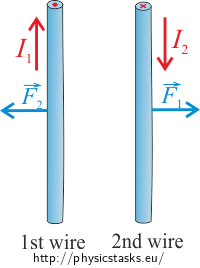• #### Summary

If parallel wires are carrying electric current, magnitudes of the forces with which they are acting on each other are equal and the following relation can be applied:

$F=\frac{\mu_o}{2\pi}\,\frac{I_1I_2}{R}l.$

Direction of the current in the wire affects only the direction of the magnetic force. If the direction of the current in wires is congruent, they attract each other, and in case of the opposite direction of the current, wires repel each other.• #### Notation and Numerical Substitution

 $$I_1\,=10\,\mathrm{A}$$ current carried by the first wire $$I_2\,=20\,\mathrm{A}$$ current carried by the second wire $$R\,=\,10\,\mathrm{cm}=0.10\,\mathrm{m}$$ distance between the wires $$l\,=\,1\,\mathrm{m}$$ length of the wire $$F\,=\,?$$ force acting on a wire of length l From the Tables: $$\mu_o=4\pi \cdot 10^{-7}\,\mathrm{Hm^{-1}}$$ permeability of vacuum

$F=\frac{\mu_o}{2\pi}\,\frac{I_1I_2}{R}\,l=\frac{4\cdot\pi \cdot 10^{-7}}{2\cdot\pi}\cdot\,\frac{10{\cdot} 20}{0.1}\cdot1\,\mathrm{N}=4{\cdot} 10^{-4}\,\mathrm{N}=0.4\,\mathrm{mN}$

The magnitude of the forces with which the wires interact with each other does not depend on the direction of the current in the wires and is, in both cases, equal to 0.4 mN.

If the direction of the current in the wires is congruent, they attract each other, and in case of the opposite directions of the currents, wires repel each other.

• #### Comment: Definition of the Ampere

Based on the obtained relation for the magnitude of the magnetic force with which wires interact with each other, the unit of current, ampere, is defined. It is one of the seven base units of the SI.

Definition of the Ampere

One ampere is a constant current which, when passing through two straight parallel infinitely long wires of a negligible cross section located in vacuum at a distance of 1 m from each other, causes a force between the wires of the magnitude of 2·10-7 N on 1 m of the length of the wire.